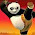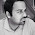Thursday, February 24, 2011

Using Bayesian Average to “Rank” Content Based on User Ratings

I recently completed the rollout of a brand new “Rating” system for our ninemsn site image content, essentially allowing users of our site to use the familiar “Star” method to rate each image in a slideshow like so:

Here, the user is about to rate this image “4 stars” and this image already has an “Average Rating” of 4.0 stars.

How is the “Average Rating” calculated?
Simple, let’s say that prior to us rating, 2 people rated this image 4 stars each, so using this simple calculation we can work it out.

Average Rating (4.0) = Total Rating (8) / Total People Who Rated (2)

So, what’s the difference between Ranking and Average Rating?
Let’s take a deeper look into this, the above mentioned rating system is used on a lot of sites like YouTube and iTunes where users are allowed to rate their content, in this case videos and music tracks. These ratings are then used to let other users discover new popular content on sites and act as a kind of “wisdom of the crowd” concept, where people tend to like content that others like.

This kind of rating based discovery of content is usually done by “Ranking” content based on the user ratings of the content, you can see this on YouTube where you can search for videos and sort it by rating.

Now this is where it gets tricky as the Ranking is a different concept than Rating, and the search results in the above YouTube search are going to show results ordered by the Ranking and not by the average rating of each video.

Let’s say we have 2 videos that have been tagged “southpark”.

Video 1:
Title: “Kenny Dies Again”:
Uploaded on: 10 Feb 2010

So far, 100 people have rated this video and it had a "Total Rating" of 300, therefore this video has an Average Rating of 3.0 stars (300/100).

Video 2:
Title: “Cartman plots to take over world”
Uploaded: 11 Feb 2011

So far, 2 people have rated this video 4 stars each and therefore a “Total Rating" of 8 which gives it an Average Rating of 4.0 stars.

So if the above search request for “southpark” returned videos based on the Average Rating value then “Cartman plots to take over world” would be given a higher priority than “Kenny Dies Again”. This is clearly wrong as a lot more people rated “Kenny Dies Again” and therefore this video should be given a higher priority.

So this is where Ranking becomes useful, ranking lets you take the above situation into consideration and give you a more accurate ordering of content based on the rating and the total votes each piece of content receives. In other words, it gives a more accurate picture on how people feel about a piece of content.
So in summary, average rating gives you an idea of how people feel about a single image whereas Raking lets you rank multiple images based on user ratings and see which is more relevant than the other.

Now that we understand the need for Ranking, how can we calculate it?
One commonly used way to do this is to use the “Bayesian Average” calculation. Wikipedia has got a good explanation of it here.

Essentially what it means is that if we look at the above “southpark” search example again, where video 2 had a larger average rating that video 1 because it got a lot less votes, we need to use some other constant value to give video 1 a higher “relevancy weight” than video 2 and hence give it a higher ranking. This “other constant value” can be anything, so long as you consider it relative to what you are trying to do and something would help us improve the “relevancy weight” and the quality of the calculation.

So in this example, because we are trying to see how a video’s rating can determine its rank compared to all other videos we have and because we know that the “more” people who rate a video essentially carries more weight, we can use this as the “other constant  value”.

Other Constant Value  = Average Number of Votes across all videos * Average Rating of all videos

This works out to be a good number to compare against as we are trying to compare our videos against all the existing rated videos. So we can use this formula to work out the Bayesian Rating of a video:

Bayesian Rating
(Average Number of Votes across all videos * Average Rating of all videos) + (Total People Who Rated * Video’s Total Rating) / (Average Number of Votes across all videos + Total People Who Rated)

So if we know that:

Average Number of Votes across all videos = 1500
Average Rating of all videos = 3.5

And we use this formula to calculate the ranking of video 1 and video 2 mentioned above:

Video 1:
Rank = (1500 * 3.5) + (100 * 300) / (1500 + 100)
Therefore, Rank = 22.03

Video 1:
Rank = (1500 * 3.5) + (2 * 8) / (1500 + 2)
Therefore, Rank = 3.5

So based on this it very clear that video 1 has a much higher “weight” than video 2 :)

The only this you need to keep in mind here is that the “other constant value” we used above (i.e. Average Number of Votes across all videos * Average Rating of all videos) needs to be constant across your ranking calculations. This value can change as obviously overtime the values for both Average Number of Votes across all videos and Average Rating of all videos are going to increase, and that’s fine, but make sure that when you calculate the rank for each content item on your site, use the same value. Confused?

Well, think of it this way. All the individual “Ranks” for your content would be stored in a separate database table (i.e. Video1 = 22.03, Video 2 = 3.5), and your searches use this table to order the search results. Now the process of calculating the Rank and keeping it updated for all your videos would be a SQL job that runs every 5 mins and step 1 of this SQL Job would be to calculate a fresh new “other constant value” and then use that to calculate and store rank for each content item in the rank table. Hopefully this makes sense now.

I’m sorry if this all sounds very confusing and to be honest I confused myself as I over analysed the Bayesian average formula, but the secret is to keep it simple and work out a good solid reusable formula that you can use on your site to determine rank based on user ratings. If you got any questions, then please ask me using the comments section below and i'll try my best to clarify.

Here is another good article that I found very useful:

Good luck and happy rating :)

1.I have recently become obsessed with this topic, and have done a lot of writing and a lot of research, but still have some unanswered questions.

Why doesn't YouTube calculate ratings in this way? When you arrange the videos by 'most liked', it doesn't take into account the ratio of likes to dislikes. I think it would be good to at least have this as an option, but I can't seem to find any easy way of accomplishing it. Any thoughts?

-Ryan

2.You are right, this is a very interesting topic indeed :) That's an interesting observation you have about how YouTube orders its videos when you select the 'most liked' filter.. I mentioned in my post that 'you use an “other constant value” to give give you a relevancy weight on content... now I believe that this can be anything you feel is a good benchmark to calculate most liked content.. so maybe YouTube uses 'dislike' counts as well to calculate this constant value... which would make sense...

3.Other Constant Value  = Average Number of Votes across all videos
NOT Average Number of Votes across all videos * Average Rating of all videos

Thanks for the great write up.

4.Once I get the Bayesian Average, How can I convert that to 5 STAR rating ? I mean If I get a large value like 616653, how to get a value relative to 5 ? Thanx in advance.

1.You already have your 5 star rating. You are just using the Bayesian average to rank the ratings in a more valid way based upon sample size.

2.You already have your 5 star rating as the simple average. You are just using the Bayesian Average to rank these ratings based upon sample size and condidence.

5.The Bayesian rating is done to rank existing rated content (video, image
etc) against each other. For example, is a search feature when the user
wants to order results based on the ratings.

But if a user is viewing a single piece of content (like what you
described) you calculate and show the average rating in the normal way.

E.g:

If a video got 2 ratings

, and the first was 3 out of 5 and the second was 2 out of 5.

The average rating out of 5 stars is

= Total rating count  / total ratings

So it's: (3+5) /2 = 4

6.Seems so simple but how to do you calculate the "Average Number of Votes across all videos"? Thanks :)

1.Hi Sam,

Bumped into this interesting post by Mark Paul and had the same question. I think the answer to your question and mine is,

Assuming your database contains 500 videos, which has a total of 2000 votes made by 1000 users today, hence the

Average Number of Votes across all videos = 2000 / 500 = 4 votes per video

HTH,
Lobbie

2.Hi Same, this is a really late reply.

But it's exactly like what lobbie said :)

3.Interesting article. I'm still confused about the "Average Number of Votes across all videos". In this context are votes = stars or reviews?

If say we had 5 items in our group. With 800 total stars earned/awarded across all items, 5 possible stars for each review, and a total of 180 reviews across all items. What would the "Average Number of Votes across all videos" be?

7.Hi.I have the same question as aranyakamal.how do I convert the Bayesian weighted scores on a scale of 1-5.

8.Hi, I believe the answer from newbreedofgeek is the answer. A suggestion I have could be you do an interpolation between the scale of 1-5 and the Bayesian weighted scores (assuming the intervals are of same distance and the relationship is linear). Eg. 1 = min Bayesian weighted score while 5 = max Bayesian weighted score so if you have a Bayesian weighted score between min and max, you can calculate the corresponding score of the 1-5 scale. Here is the formula http://www.ajdesigner.com/phpinterpolation/linear_interpolation_equation.php

9.@lobbie lobbie. Thanks for that . Also, shoudlnt the avg_num_votes for all videos in this case be (100+1)/2= 50.5. How did 1500 come into picture here?

10.@lobbie lobbie. Thanks for that . Also, shoudlnt the avg_num_votes for all videos in this case be (100+2)/2= 51. How did 1500 come into picture here?